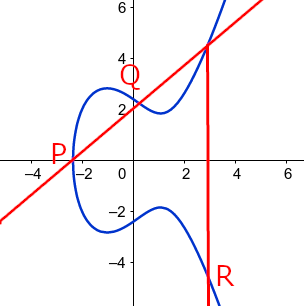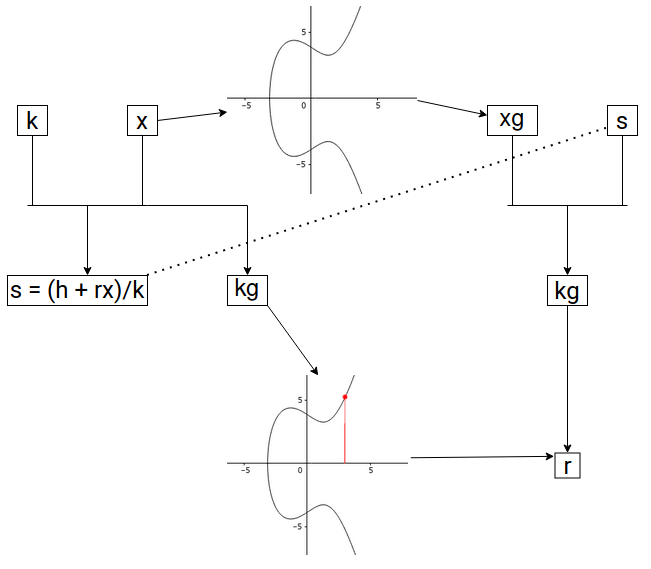# Intro to Elliptic Curve Cryptography

This document introduces the fundamental math behind elliptic curves, and the ideas behind how this math can be used in cryptography. Then, this document discusses elliptic curve signatures, and explains the significance behind BLS signatures.

## One-way Functions and Private/Public Keys

One of the big ideas of cryptography is that there are certain `one-way' functions which, given an input, is easy to compute an output for, but is much more difficult to do the reverse. These allow secure information transfer information. As a basic example, suppose you have a bucket of blue paint. Then, one such function might take an input in the form of a bucket of paint of some color, and mixing it with the blue paint. It’s easy for you to do so and create an output of a different color, but it will be hard to take this output and separate the two paints.

This is where elliptic curves come in. An elliptic curve is an equation of the form y^2 = ax^3 + bx + c.Note that the curve is symmetric about the x-axis due to the y^2 term. Given two points P, Q on such a curve, the line crossing through them is guaranteed to hit a third point on the curve. In the edge case that this line is vertical, one can say that there is a point at infinity at which all vertical lines intersect, and in the case that P = Q, one takes the tangent line, which will again only intersect the curve one more time. If you take this third point and reflect it over the x-axis to get R, and define P + Q = R according to this process, then + is a group operation on this elliptic curve.

Being a group operation means that this binary operation satisfies certain properties; namely, (a + b) + c = a + (b + c), there exists an identity element P (in this case, the point at infinity) that satisfies P + a = a + P = a (think adding zero), and for every element a, there is an inverse element -a, such that a + (-a) = P. To put it simply, one can use + in similar ways as regular addition.

What this means is that one can create an especially nice one-way function. First, let’s take the points on an elliptic curve modulo a prime; then, choose a generator g, which, when added to itself enough times, runs through all points; then, given an input of integer x, define the output as g + g + … + g = xg.

If you recall the operation, xg is just straightforward algebra to compute. One can speed it up by thinking about x in binary; to compute 12g for example, first compute 2g, then 4g, 8g, and finally 12g. This is allowed by group properties.

Yet, given xg and g, it becomes extremely hard to compute x. In fact, one can’t do that much better than just multiplying g over and over and hoping to get xg. This is called the elliptic curve discrete logarithm problem (ECDLP).

Thus, it makes sense for one to create a secret key x, and set their public key as xg. In this way, no one can compute the secret key given the public key. However, one can use the secret key to show others that knowledge the secret key, without actually showing the secret key; in other words, securely proving the identity. Due to how secure ECDLP is, the amount of valuable resources saved by how efficient and compact elliptic curves can be compared to other systems, and other algebraic properties of this curve that become important in the upcoming algorithms, ECC has become the method of choice by most cryptocurrencies.

## Elliptic Curve Signatures

### ECDSA

To see how ECC is implemented, let’s look at the problem of signatures. We want to create a method so that a person can generate signatures which can be used to verify that person’s consent, but it must be so that everybody else can verify that the signature did indeed come from that person, and it must be infeasible (such as being equivalent to solving ECDLP) to replicate another person’s signature.

To get an idea of how this is possible, consider using your secret information to generate some kind of output, and publishing enough information so that one can compute the same output in a different manner using the published information; however, having the secret information be unrecoverable given the published information. In this way, we can use this output and published information as a signature, so that others can use this computation to verify it, without knowing the secret information used to create it in the first place.

In the Elliptic Curve Discrete Signature Algorithm (ECDSA)’s case, suppose one wishes to sign a message with secret key x and public key xg. First, generate a random number k and compute R = kg, and let r be the x-coordinate of this point. Then, for a hashed message h, compute s = (h + rx) / k mod p. The signature is (r, s).The main idea outlined before is present here; using this secret k, you can created R = kg, which can be computed in two different ways. To see this, for verification, use the published information h, r, s, and xg. Then, compute P = (h/s)g + (r/s)xg = (h + rx)g/s = kg = R. Finally, using R, one can recover the x-coordinate r, and verify it’s indeed in (r, s). Although verification is straightforward, to replicate the signature (r, s) is infeasible without knowledge of k and x.

### BLS Signatures

However, in the case that multiple signatures are required, there arises an issue of computation and space. Specifically, suppose you want to collect several signatures from different nodes for some message. Then, you must collect each individual’s signature, and verify them one-by-one. Boneh-Lynn-Shacham (BLS) signatures solves this issue. This algorithm still follows the main idea outlined before; however, by using properties unique to elliptic curves (whose details are omitted for the sake of brevity, but available in the DKG/BLS documentation), you are able to generate quickly computable, compact, and aggregatable signatures. Given signatures s_1, s_2, …, s_n, you can combine them together to generate an aggregated signature s_(n+1) of the same size. You only need to store and verify this single signature to check that all n signatures are valid.

Other advantages include being deterministic, so you don’t run into the issue of having the same signature that may be present in ECDSA, and being significantly more compact for the same level of security in the case of just one signature as compared to signatures seen in ECDSA, RSA, etc. Hence, in use cases where space and computation are expensive, BLS signatures become advantageous. To see further details of BLS, see the DKG/BLS documentation.